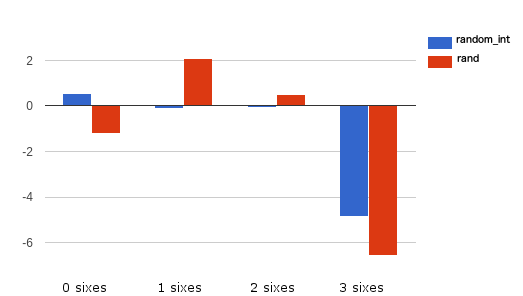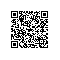# PHP 中的随机数——你觉得可靠么?## 什么是 CSPRNG？

CSPRNG 主要用于：

• 生成键（比如：生成复杂的键）
• 为新的用户账号生成随机密码
• 加密系统

## PHP 7 中的 CSPRNG

PHP 7 为 CSPRNG 引入了两种新函数：random_bytesrandom_int

random_bytes 函数返回 string 类型，并接受一个 int 类型为参数，该参数规定了所返回字符串的字节长度。

$bytes = random_bytes('10'); var_dump(bin2hex($bytes));
//possible ouput: string(20) "7dfab0af960d359388e6"  

random_int 函数返回给定范围内的整型数字。

var_dump(random_int(1, 100));
//possible output: 27

## 幕后解密

• 在 Windows 系统，会使用 CryptGenRandom() 函数。
• 在其他平台，会优先使用 arc4random_buf() 函数（限 BSD 衍生系统或带 libbsd 的系统）。
• 若以上两点均不符合，会使用 Linux getrandom(2) 系统调用。
• 若以上来源均不符合，会抛出 Error

## 一个简例

• 0 次6 = 57.9 次
• 1 次6 = 34.7 次
• 2 次6 = 6.9 次
• 3 次6 = 0.5 次

$times = 1000000;$result = [];
for ($i=0;$i<$times;$i++){
$dieRoll = array(6 => 0); //initializes just the six counting to zero$dieRoll[roll()] += 1; //first die
$dieRoll[roll()] += 1; //second die$dieRoll[roll()] += 1; //third die
$result[$dieRoll] += 1; //counts the sixes
}
function roll(){
return random_int(1,6);
}
var_dump($result); 用 PHP 7 的 random_int 与简单的 rand 函数测试上面的代码，可能会得到： Sixes expected random_int rand 0 579000 579430 578179 1 347000 346927 347620 2 69000 68985 69586 3 5000 4658 4615 更直观地查看 randrandom_int 的差别，可以运用方程式放大两组结果的差异，并绘制成图表： php result - expected result / sqrt(expected) 得到的结果如下：(结果越接近零越好) 即便三个6的组合表现一般，且该测试与真实应用相比太过简单，我们也能清楚地看到 random_int 的表现优于 rand。况且，随机数生成器的可预见行为、重复行为越少，应用的安全程度就更高。 ## PHP 5 又如何呢？ 默认情况下，PHP 5 并未提供任何强虚拟随机数生成器。而实际使用中，可以使用 openssl_random_pseudo_bytes()mcrypt_create_iv() 方法，或直接结合使用 /dev/random/dev/urandomfread() 方法。此外，还有包 RandomLiblibsodium 如果你想用一个比较好的随机数生成器，同时能与 PHP 7 兼容，你可以使用 Paragon Initiative 公司的 random_compat 库。该库允许在 PHP 5.x 项目中使用 random_bytes()random_int() 方法。 该库可以使用 Composer 进行安装： composer require paragonie/random_compat require 'vendor/autoload.php';$string = random_bytes(32);
var_dump(bin2hex($string)); // string(64) "8757a27ce421b3b9363b7825104f8bc8cf27c4c3036573e5f0d4a91ad2aaec6f"$int = random_int(0,255);
var_dump($int); // int(81) random_compat 库使用了与 PHP 7 中不同的优先序列： 1. 如果可用，先使用 fread() /dev/urandom 2. mcrypt_create_iv($bytes, MCRYPT_CREATE_IV)
3. COM('CAPICOM.Utilities.1')->GetRandom()
4. openssl_random_pseudo_bytes()

$passwordChar = '0123456789abcdefghijklmnopqrstuvwxyzABCDEFGHIJKLMNOPQRSTUVWXYZ';$passwordLength = 8;
$max = strlen($passwordChar) - 1;
$password = ''; for ($i = 0; $i <$passwordLength; ++$i) {$password .= $passwordChar[random_int(0,$max)];
}
//possible output: 7rgG8GHu

## 总结

OneAPM for PHP 能够深入到所有 PHP 应用内部完成应用性能管理 能够深入到所有 PHP 应用内部完成应用性能管理和监控，包括代码级别性能问题的可见性、性能瓶颈的快速识别与追溯、真实用户体验监控、服务器监控和端到端的应用性能管理。想阅读更多技术文章，请访问 OneAPM 官方技术博客